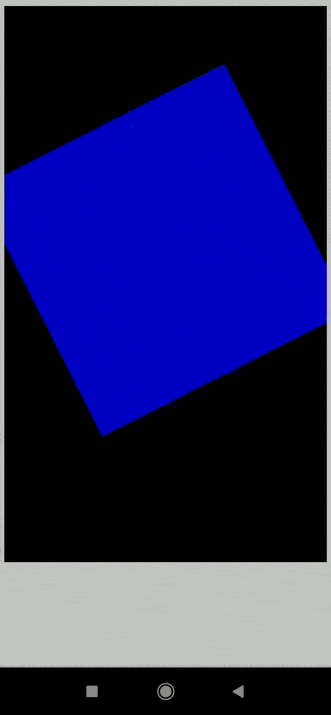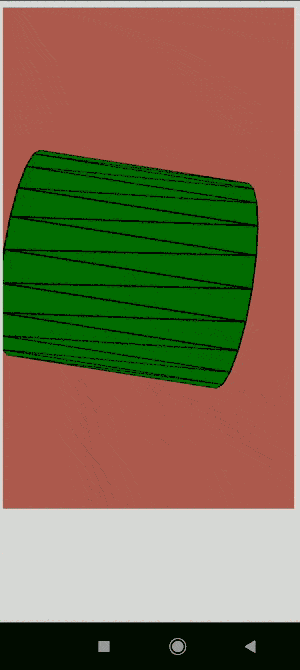# p5.js rotationZ Event

• Last Updated : 13 Jul, 2022

The system variable rotationZ in p5.js is responsible for the rotation of mobile devices (smartphones and tablets) always along the z-axis. It can be used in the draw() function to continuously get the current rotation along the z-axis. If the graphics angleMode() is set to DEGREES, then the value will be in the range of 0 to 360. When it is set to RADIANS, the value will be 0 to 2*PI. This variable is only available for devices with a built-in compass.

Note: The order the rotations are called is important if all the three variables are used together. It is required to call them in the order Z, Y, and X to prevent inconsistencies.

Syntax:

`rotationZ`

Example 1:

## Javascript

 `// Rotate the device to see the box move` `function` `setup() {` `  ``createCanvas(600, 600, WEBGL);` `}`   `function` `draw() {` `  ``background(2);`   `  ``// Set the rotation to be equal to` `  ``// the variable rotationZ` `  ``rotateZ(radians(rotationZ));`   `  ``fill(``"blue"``);` `  ``box(200, 200, 200);` `}`

Output:Example 2:

## Javascript

 `function` `setup() {` `  ``createCanvas(600, 600, WEBGL);` `}`   `function` `draw() {` `  ``background(205, 105, 94);` `  `  `  ``// Set the rotation to be equal to` `  ``// the variable rotationZ` `  ``rotateZ(radians(rotationZ));` `  `  `  ``fill(``'green'``);` `  ``cylinder(100, 200);` `}`

Output:My Personal Notes arrow_drop_up
Recommended Articles
Page :